Next: A more robust smoothness Up: Smoothness estimation Previous: Smoothness estimation

### Smoothness a la SPM

The current implementations of SPM (SPM99) use the smoothness estimation as described in Kiebel et al .

For a Gaussian random field the smoothness is defined as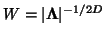where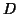is the dimensionality of the field and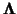the covariance matrix of it's first partial derivatives. An unbiased estimator for the covariance of the partial derivatives is given by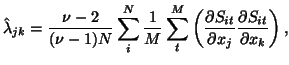(10)

where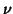is the number of degrees of freedom,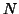the number of voxel positions (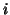) and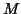the number of time points (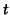) in a FMRI time series. In fact we don't have to evaluate this equation for the whole covariance matrix. The off-diagonal elements will be zero so we only need to calculate the diagonal elements of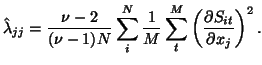(11)

The partial derivative is evaluated via the gradient operator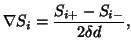(12)

for non-edge voxels, where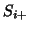and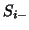are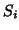s neighbouring voxels in the dimension along which the derivative is currently being evaluated.

Now we can calculate the FWHM of the theoretical Gaussian responsible for the observed smoothness(13)

where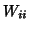is the variance,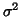, of the Gaussian point-spread-function computed from the covariance measure for theth dimension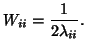(14)

A combined value for the FWHM is given by the geometric mean of the various FWHM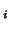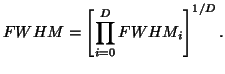(15)Next: A more robust smoothness Up: Smoothness estimation Previous: Smoothness estimation
David Flitney 2001-11-29